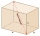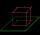# Space diagonal + area - math problems

#### Number of problems found: 27

• Regular square prismThe volume of a regular square prism is 192 cm3. The size of its base edge and the body height is 1: 3. Calculate the surface of the prism.
• Cuboid diagonalsThe cuboid has dimensions of 15, 20 and 40 cm. Calculate its volume and surface, the length of the body diagonal and the lengths of all three wall diagonals.The height of a regular quadrilateral prism is v = 10 cm, the deviation of the body diagonal from the base is 60°. Determine the length of the base edges, the surface, and the volume of the prism.
• Regular hexagonal prismCalculate the volume of a regular hexagonal prism whose body diagonals are 24cm and 25cm long.Calculate the volume (V) and the surface (S) of a regular quadrilateral prism whose height is 28.6 cm and the deviation of the body diagonal from the base plane is 50°.
• Space diagonalThe space diagonal of a cube is 129.91 mm. Find the lateral area, surface area and the volume of the cube.
• Height of the roomGiven the floor area of a room as 24 feet by 48 feet and space diagonal of a room as 56 feet. Can you find the height of the room?
• Prism diagonalThe body diagonal of a regular square prism has an angle of 60 degrees with the base, the edge length is 10 cm. What is the volume of the prism?
• Cube diagonalsThe cube has a wall area of 81 cm square. Calculate the length of its edge, wall, and body diagonal.
• Body diagonalCalculate the volume and surface of the cube if the diagonal body measures ten dm.
• Solid cuboidA solid cuboid has a volume of 40 cm3. The cuboid has a total surface area of 100 cm squared. One edge of the cuboid has a length of 2 cm. Find the length of a diagonal of the cuboid. Give your answer correct to 3 sig. Fig.
• Cube cutThe cube ABCDA'B'C'D ' has an edge of 12cm. Calculate the area of diagonal cut B DD'B '.
• Body diagonal - cubeCalculate the surface and cube volume with body diagonal 15 cm long.
• Right triangular prismWe have a cuboid with a base and dimensions of 12 cm and 5 cm and a height of 4 cm. The tablecloth is cut into two identical triangular prisms with right triangular bases. We painted the surface of the created prisms with color. Calculate the surface area
• Ratio-cuboidThe lengths of the edges of the cuboid are in the ratio 2: 3: 6. Its body diagonal is 14 cm long. Calculate the volume and surface area of the cuboid.
• Cube wallThe perimeter of one cube wall is 120 meters. Calculate the surface area and the body diagonal of this cube.
• Cube wallCalculate the cube's diagonal if you know that one wall's surface is equal to 36 centimeters square. Please also calculate its volume.
• Nice prismCalculate the cuboid's surface if the sum of its edges is a + b + c = 19 cm and the body diagonal size u = 13 cm.
• Four sided prismCalculate the volume and surface area of a regular quadrangular prism whose height is 28.6cm and the body diagonal forms a 50-degree angle with the base plane.
• Body diagonalThe cuboid has a volume of 32 cm3. Its side surface area is double as one of the square bases. What is the length of the body diagonal?

Do you have an exciting math question or word problem that you can't solve? Ask a question or post a math problem, and we can try to solve it.

We will send a solution to your e-mail address. Solved examples are also published here. Please enter the e-mail correctly and check whether you don't have a full mailbox.

Space diagonal - math problems. Area - math problems.